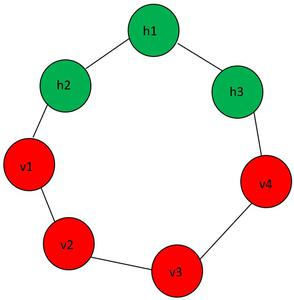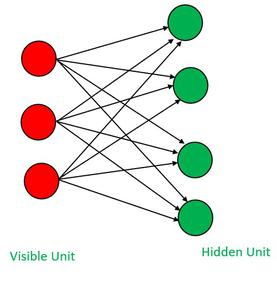Open in App
Not now

# Restricted Boltzmann Machine

• Difficulty Level : Medium
• Last Updated : 18 Mar, 2023

Introduction :

Restricted Boltzmann Machine (RBM) is a type of artificial neural network that is used for unsupervised learning. It is a type of generative model that is capable of learning a probability distribution over a set of input data.

RBM was introduced in the mid-2000s by Hinton and Salakhutdinov as a way to address the problem of unsupervised learning. It is a type of neural network that consists of two layers of neurons – a visible layer and a hidden layer. The visible layer represents the input data, while the hidden layer represents a set of features that are learned by the network.

The RBM is called “restricted” because the connections between the neurons in the same layer are not allowed. In other words, each neuron in the visible layer is only connected to neurons in the hidden layer, and vice versa. This allows the RBM to learn a compressed representation of the input data by reducing the dimensionality of the input.

The RBM is trained using a process called contrastive divergence, which is a variant of the stochastic gradient descent algorithm. During training, the network adjusts the weights of the connections between the neurons in order to maximize the likelihood of the training data. Once the RBM is trained, it can be used to generate new samples from the learned probability distribution.

RBM has found applications in a wide range of fields, including computer vision, natural language processing, and speech recognition. It has also been used in combination with other neural network architectures, such as deep belief networks and deep neural networks, to improve their performance.

What are Boltzmann Machines?

It is a network of neurons in which all the neurons are connected to each other. In this machine, there are two layers named visible layer or input layer and hidden layer. The visible layer is denoted as v and the hidden layer is denoted as the h. In Boltzmann machine, there is no output layer. Boltzmann machines are random and generative neural networks capable of learning internal representations and are able to represent and (given enough time) solve tough combinatoric problems.

The Boltzmann distribution (also known as Gibbs Distribution) which is an integral part of Statistical Mechanics and also explain the impact of parameters like Entropy and Temperature on the Quantum States in Thermodynamics. Due to this, it is also known as Energy-Based Models (EBM). It was invented in 1985 by Geoffrey Hinton, then a Professor at Carnegie Mellon University, and Terry Sejnowski, then a Professor at Johns Hopkins UniversityWhat are Restricted Boltzmann Machines (RBM)?

A restricted term refers to that we are not allowed to connect the same type layer to each other. In other words, the two neurons of the input layer or hidden layer can’t connect to each other. Although the hidden layer and visible layer can be connected to each other.

As in this machine, there is no output layer so the question arises how we are going to identify, adjust the weights and how to measure the that our prediction is accurate or not. All the questions have one answer, that is Restricted Boltzmann Machine.

The RBM algorithm was proposed by Geoffrey Hinton (2007), which learns probability distribution over its sample training data inputs. It has seen wide applications in different areas of supervised/unsupervised machine learning such as feature learning, dimensionality reduction, classification, collaborative filtering, and topic modeling.

Consider the example movie rating discussed in the recommender system section.

Movies like Avengers, Avatar, and Interstellar have strong associations with the latest fantasy and science fiction factor. Based on the user rating RBM will discover latent factors that can explain the activation of movie choices. In short, RBM describes variability among correlated variables of input dataset in terms of a potentially lower number of unobserved variables.

The energy function is given byApplications of Restricted Boltzmann Machine

Restricted Boltzmann Machines (RBMs) have found numerous applications in various fields, some of which are:

• Collaborative filtering: RBMs are widely used in collaborative filtering for recommender systems. They learn to predict user preferences based on their past behavior and recommend items that are likely to be of interest to the user.
• Image and video processing: RBMs can be used for image and video processing tasks such as object recognition, image denoising, and image reconstruction. They can also be used for tasks such as video segmentation and tracking.
• Natural language processing: RBMs can be used for natural language processing tasks such as language modeling, text classification, and sentiment analysis. They can also be used for tasks such as speech recognition and speech synthesis.
• Bioinformatics: RBMs have found applications in bioinformatics for tasks such as protein structure prediction, gene expression analysis, and drug discovery.
• Financial modeling: RBMs can be used for financial modeling tasks such as predicting stock prices, risk analysis, and portfolio optimization.
• Anomaly detection: RBMs can be used for anomaly detection tasks such as fraud detection in financial transactions, network intrusion detection, and medical diagnosis.
• It is used in Filtering.
• It is used in Feature Learning.
• It is used in Classification.
• It is used in Risk Detection.
• It is used in Business and Economic analysis.

How do Restricted Boltzmann Machines work?

In RBM there are two phases through which the entire RBM works:

1st Phase: In this phase, we take the input layer and using the concept of weights and biased we are going to activate the hidden layer. This process is said to be Feed Forward Pass. In Feed Forward Pass we are identifying the positive association and negative association.

Feed Forward Equation:

• Positive Association — When the association between the visible unit and the hidden unit is positive.
• Negative Association — When the association between the visible unit and the hidden unit is negative.

2nd Phase: As we don’t have any output layer. Instead of calculating the output layer, we are reconstructing the input layer through the activated hidden state. This process is said to be Feed Backward Pass. We are just backtracking the input layer through the activated hidden neurons. After performing this we have reconstructed Input through the activated hidden state. So, we can calculate the error and adjust weight in this way:

Feed Backward Equation:

• Error = Reconstructed Input Layer-Actual Input layer
• Adjust Weight = Input*error*learning rate (0.1)

After doing all the steps we get the pattern that is responsible to activate the hidden neurons. To understand how it works:

Let us consider an example in which we have some assumption that V1 visible unit activates the h1 and h2 hidden unit and V2 visible unit activates the h2 and h3 hidden. Now when any new visible unit let V5 has come into the machine and it also activates the h1 and h2 unit. So, we can back trace the hidden units easily and also identify that the characteristics of the new V5 neuron is matching with that of V1. This is because V1 also activated the same hidden unit earlier.Restricted Boltzmann Machines

Types of RBM :

There are mainly two types of Restricted Boltzmann Machine (RBM) based on the types of variables they use:

1. Binary RBM: In a binary RBM, the input and hidden units are binary variables. Binary RBMs are often used in modeling binary data such as images or text.
2. Gaussian RBM: In a Gaussian RBM, the input and hidden units are continuous variables that follow a Gaussian distribution. Gaussian RBMs are often used in modeling continuous data such as audio signals or sensor data.

Apart from these two types, there are also variations of RBMs such as:

1. Deep Belief Network (DBN): A DBN is a type of generative model that consists of multiple layers of RBMs. DBNs are often used in modeling high-dimensional data such as images or videos.
2. Convolutional RBM (CRBM): A CRBM is a type of RBM that is designed specifically for processing images or other grid-like structures. In a CRBM, the connections between the input and hidden units are local and shared, which makes it possible to capture spatial relationships between the input units.
3. Temporal RBM (TRBM): A TRBM is a type of RBM that is designed for processing temporal data such as time series or video frames. In a TRBM, the hidden units are connected across time steps, which allows the network to model temporal dependencies in the data.

My Personal Notes arrow_drop_up